@Team 2019-04-08T06:57:39.000000Z 字数 7027 阅读 1166

# 大业14年

石文华

## 1、FCN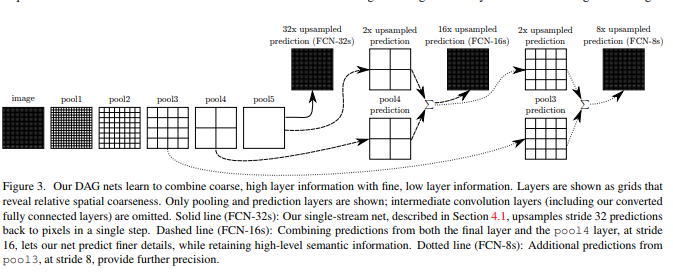1、32x upsampled prediction

2、16x upsampled prediction

3、8x upsampled prediction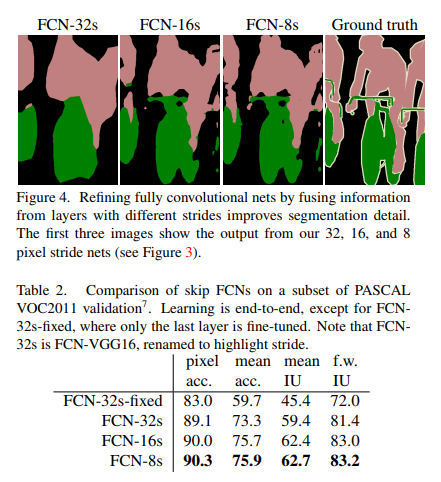## 2、SegNet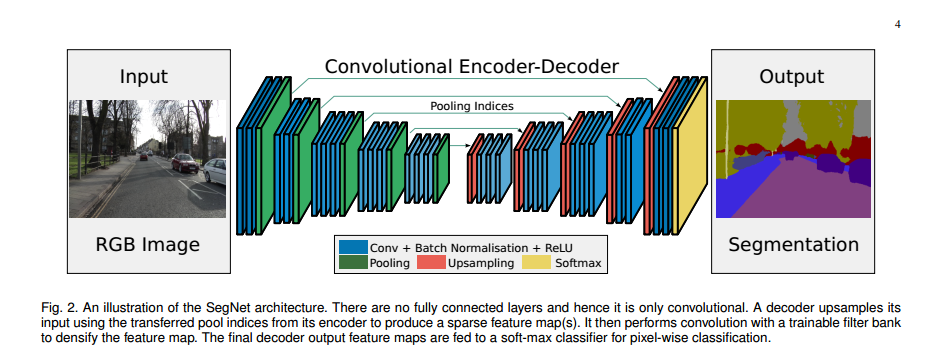### 编码和解码过程

SegNet在编码阶段进行pooling的时候会保留保存通过max选出的权值在2x2 filter中的相对位置，用于之后在解码器中使用那些存储的索引来对相应特征图进行去池化操作，如下图所示：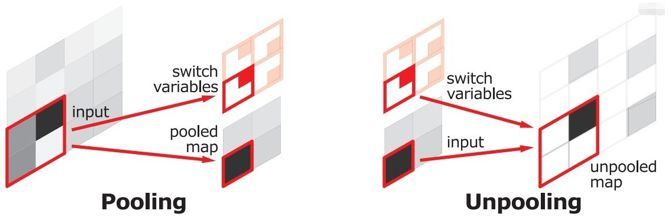## 3、U-net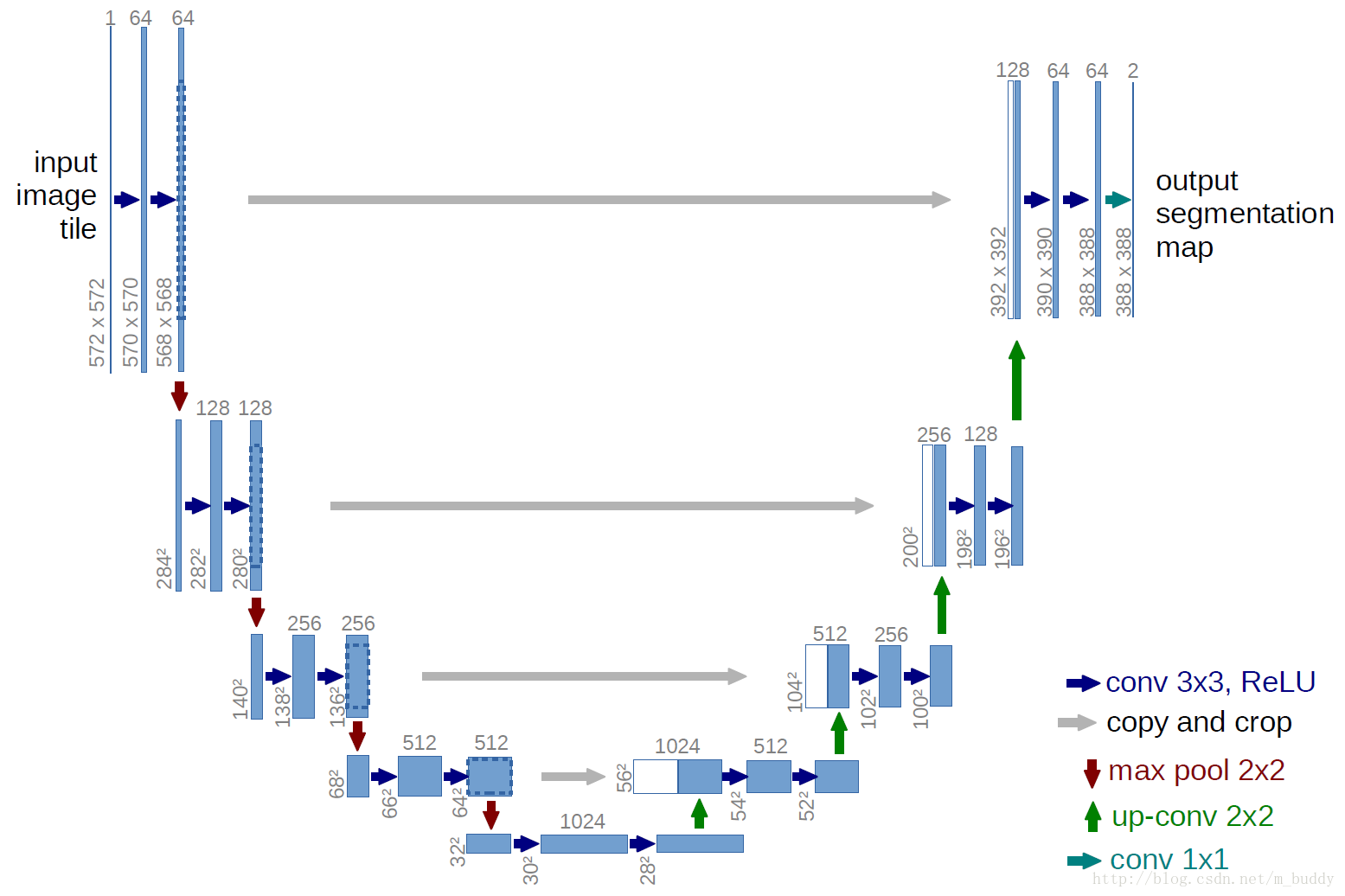1、下采样（Conv+maxpooling），即收缩路径,分辨率减小，通道数增加，位置信息变少，语义信息增强。因此，较浅的高分辨率层（位置信息强的层）的特征可以用来解决像素定位的问题，较深的层（语义信息强的层）的特征可用来解决像素分类的问题。
2、上采样（反卷积），即扩张路径，增加输出的分辨率。上采样之后需要增加下采样中的高分辨率特征(high resolution features)，采用的是叠加（concatenate操作）的做法，而不是FCN中的横向连接（加）操作。这样综合的特征将使得结果更加精确。
3、扩张路径基本对称于收缩路径，只是网络在上采样部分依然有大量的特征通道(feature channels)，只需要每次使用反卷积的时候都将特征通道数量减半，特征图大小加倍。这样能将特征信息向更高的分辨率层(higher resolution layers)传播。

"""Spyder EditorThis is a temporary script file."""import numpy as npimport osimport skimage.io as iofrom keras.models import Modelfrom keras.layers import Conv2D,Input,MaxPooling2D,Dropout,concatenate,UpSampling2Dfrom keras.optimizers import *from keras.callbacks import ModelCheckpoint,LearningRateSchedulerfrom keras import backend as kerasinput_shape=(256,256,1)#定义输入inputs=Input(input_shape)conv1=Conv2D(64,3,activation='relu',padding='same',kernel_initializer='he_normal')(inputs)conv1=Conv2D(64,3,activation='relu',padding='same',kernel_initializer='he_normal')(conv1)pool1=MaxPooling2D(pool_size=(2,2))(conv1)conv2=Conv2D(128,3,activation='relu',padding='same',kernel_initializer = 'he_normal')(pool1)conv2=Conv2D(128,3,activation='relu',padding='same',kernel_initializer='he_normal')(conv2)pool2=MaxPooling2D(pool_size=(2,2))(conv2)conv3=Conv2D(256,3,activation='relu',padding='same',kernel_initializer='he_normal')(pool2)conv3=Conv2D(256,3,activation='relu',padding='same',kernel_initializer='he_normal')(conv3)pool3=MaxPooling2D(pool_size=(2,2))(conv3)conv4=Conv2D(512,3,activation='relu',padding='same',kernel_initializer='he_normal')(pool3)conv4=Conv2D(512,3,activation='relu',padding='same',kernel_initializer='he_normal')(conv4)drop4=Dropout(0.5)(conv4)pool4=MaxPooling2D(pool_size=(2,2))(drop4)conv5=Conv2D(1024,3,activation='relu',padding='same',kernel_initializer='he_normal')(pool4)conv5=Conv2D(1024,3,activation='relu',padding='same',kernel_initializer='he_normal')(conv5)drop5=Dropout(0.5)(conv5)up6=Conv2D(512,2,activation='relu',padding='same',kernel_initializer = 'he_normal')(UpSampling2D(size=(2,2))(drop5))merge6=concatenate([drop4,up6],axis=3)conv6 = Conv2D(512, 3, activation = 'relu', padding = 'same', kernel_initializer = 'he_normal')(merge6)conv6 = Conv2D(512, 3, activation = 'relu', padding = 'same', kernel_initializer = 'he_normal')(conv6)up7 = Conv2D(256, 2, activation = 'relu', padding = 'same', kernel_initializer = 'he_normal')(UpSampling2D(size = (2,2))(conv6))merge7 = concatenate([conv3,up7], axis = 3)conv7 = Conv2D(256, 3, activation = 'relu', padding = 'same', kernel_initializer = 'he_normal')(merge7)conv7 = Conv2D(256, 3, activation = 'relu', padding = 'same', kernel_initializer = 'he_normal')(conv7)up8 = Conv2D(128, 2, activation = 'relu', padding = 'same', kernel_initializer = 'he_normal')(UpSampling2D(size = (2,2))(conv7))merge8 = concatenate([conv2,up8], axis = 3)conv8 = Conv2D(128, 3, activation = 'relu', padding = 'same', kernel_initializer = 'he_normal')(merge8)conv8 = Conv2D(128, 3, activation = 'relu', padding = 'same', kernel_initializer = 'he_normal')(conv8)up9 = Conv2D(64, 2, activation = 'relu', padding = 'same', kernel_initializer = 'he_normal')(UpSampling2D(size = (2,2))(conv8))merge9 = concatenate([conv1,up9], axis = 3)conv9 = Conv2D(64, 3, activation = 'relu', padding = 'same', kernel_initializer = 'he_normal')(merge9)conv9 = Conv2D(64, 3, activation = 'relu', padding = 'same', kernel_initializer = 'he_normal')(conv9)conv9 = Conv2D(2, 3, activation = 'relu', padding = 'same', kernel_initializer = 'he_normal')(conv9)conv10 = Conv2D(1, 1, activation = 'sigmoid')(conv9)model = Model(input = inputs, output = conv10)model.summary()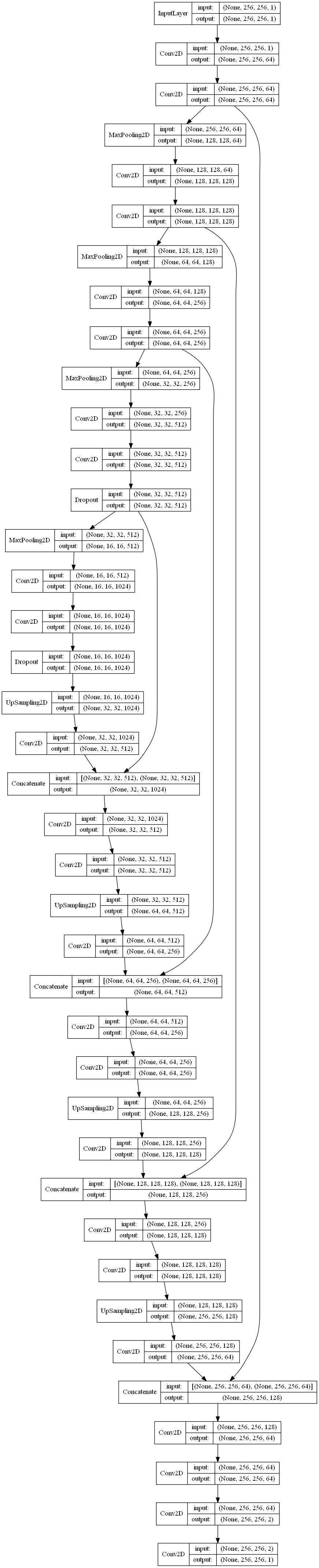## 4、Fully Convolutional DenseNet

### 1、前言：

DenseNet 是一种具有密集连接的卷积神经网络，即任何两层之间都有直接的连接，也就是说，网络每一层的输入都是前面所有层输出的并集，而该层所学习的特征图也会被直接传给其后面所有层作为输入。这种密集连接通过将特征在channel上的连接来实现特征重用（feature reuse），这让DenseNet在参数和计算成本更少的情形下实现比ResNet更优的性能，DenseNet也因此斩获CVPR 2017的最佳论文奖。

### 2、密集连接机制：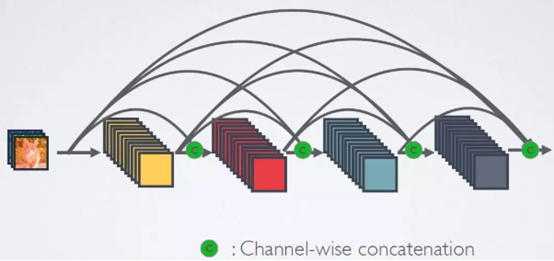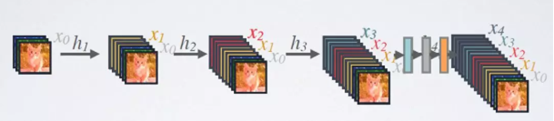### 3、网络组成架构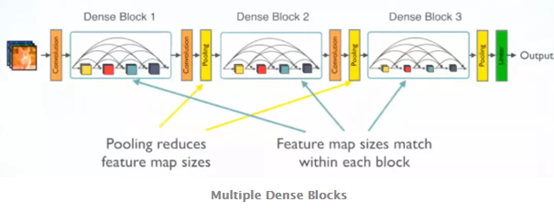### 4、DenseBlock和Transition的细节

（1）非线性组合：
DenseBlock中是非线性组合函数，比如BN+ReLU+3x3 Conv结构，而且各个层的特征图大小是一致的，这样才能保证在channel维度上连接。
（2）增长率（Growth rate）：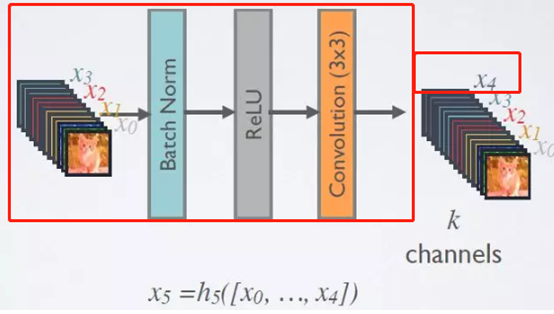（3）bottleneck层：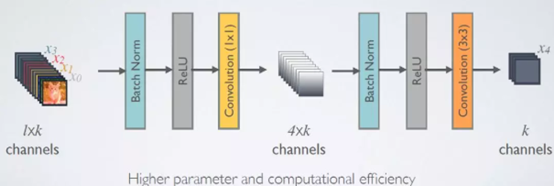（4）Transition层：
Transition层也是非线性变换函数组成的模块，组合方式为：BN+ReLU+1x1 Conv+2x2 AvgPooling，既然是非线性变换组合，在这个组合中加入pooling就可以起到降低特征图大小的作用，那么它也可以通过控制输入的卷积核个数，起到进一步压缩特征图的作用，从而进一步优化模型。假设一个Denseblock中包含m个特征图,那么我们使其输出连接的transition layer层生成⌊θm⌋个输出特征图.当这里的θ取值为（0,1]时，起到压缩的作用，因此可以叫做压缩系数, 当θ=1时,transition layer将保留原feature维度不变。

### 5、DenseNet 的优点

(1)省参数, 在 ImageNet 分类数据集上达到同样的准确率，DenseNet 所需的参数量不到 ResNet 的一半
(2)省计算。达到与 ResNet 相当的精度，DenseNet 所需的计算量也只有 ResNet 的一半左右.
(3)抗过拟合, 对于 DenseNet 抗过拟合的原因有一个比较直观的解释：神经网络每一层提取的特征都相当于对输入数据的一个非线性变换，而随着深度的增加，变换的复杂度也逐渐增加（更多非线性函数的复合）。相比于一般神经网络的分类器直接依赖于网络最后一层（复杂度最高）的特征，DenseNet 可以综合利用浅层复杂度低的特征，因而更容易得到一个光滑的具有更好泛化性能的决策函数。
(4)支持特征重用，强化特征传播，有效解决梯度消失问题。

### 6、FC-DenseNet语意分割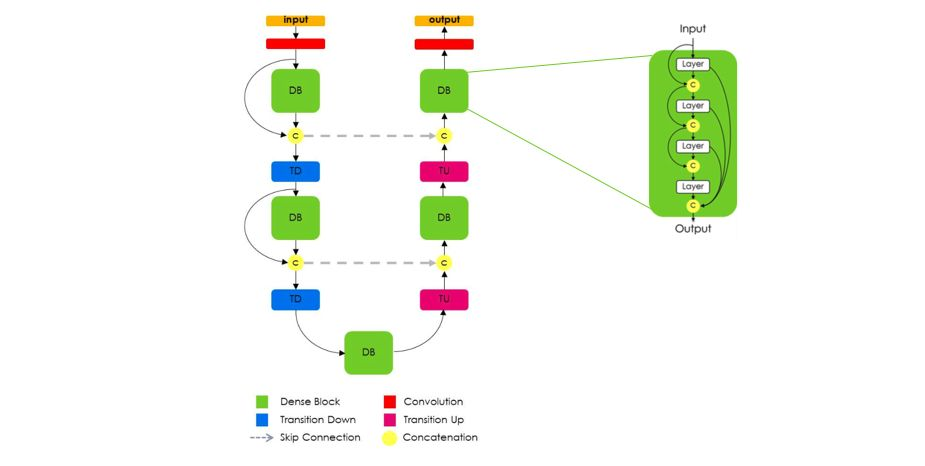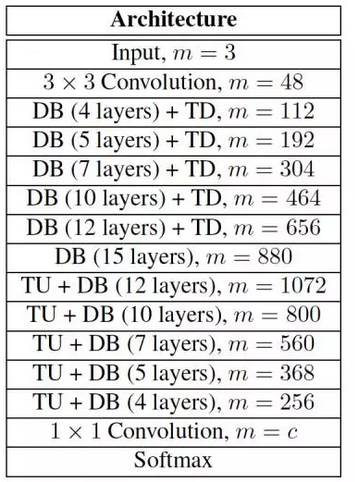## 5、 DeepLab系列• 私有
• 公开
• 删除